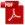### 15.2.8. Buckling of Triangular Panels

Reference: Abbott, Richard. Analysis and Design of Composite and Metallic Flight Vehicle Structures 3 Edition, 2019.

15.2.8.1. Compression Buckling of Triangular Panels

This method is briefly covered in the excellent general reference:

(NACA-TN-3781, 1957). There is little information given but it is worth including in this text.

General curve fits have been done for the curves in Figure 15.2.8‑1.

For the simply supported triangular panel the following expression can be used to calculate k:

And for the clamped edge condition the following expression can be used:

These approximations are available in this spreadsheet:

The values for k can be used in the classic buckling expression where b is the ‘base’ of the isosceles triangle:

There is no information available at this time on the plastic buckling of triangular panels in compression. It is advised to account for panel material plasticity, if Fcr    exceeds Fcy then limit Fcr  to Fcy . The buckling compression buckling analysis spreadsheet link is below:

15.2.8.2. Shear Buckling of Triangular Panels

As before, this method is briefly covered in the excellent general reference  (NACA-TN-3781, 1957):

General curve fits have been done for the curves in Figure 15.2.8‑3:

For the simply supported triangular Panel the following expression can be used to calculate k:

And for the clamped edge condition the following expression can be used:

These approximations are available in this spreadsheet:

The values for k can be used in the classic buckling expression where b is the ‘base’ of the isosceles triangle.

### 15.2.8. Buckling of Triangular Panels

Reference: Abbott, Richard. Analysis and Design of Composite and Metallic Flight Vehicle Structures 3 Edition, 2019.

15.2.8.1. Compression Buckling of Triangular Panels

This method is briefly covered in the excellent general reference:

(NACA-TN-3781, 1957). There is little information given but it is worth including in this text.

General curve fits have been done for the curves in Figure 15.2.8‑1.

For the simply supported triangular panel the following expression can be used to calculate k:

And for the clamped edge condition the following expression can be used:

These approximations are available in this spreadsheet:

The values for k can be used in the classic buckling expression where b is the ‘base’ of the isosceles triangle:

There is no information available at this time on the plastic buckling of triangular panels in compression. It is advised to account for panel material plasticity, if Fcr    exceeds Fcy then limit Fcr  to Fcy . The buckling compression buckling analysis spreadsheet link is below:

15.2.8.2. Shear Buckling of Triangular Panels

As before, this method is briefly covered in the excellent general reference  (NACA-TN-3781, 1957):

General curve fits have been done for the curves in Figure 15.2.8‑3: